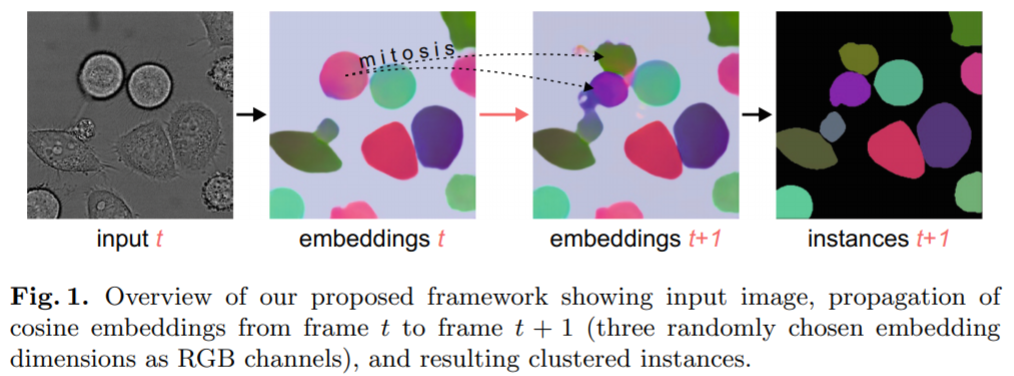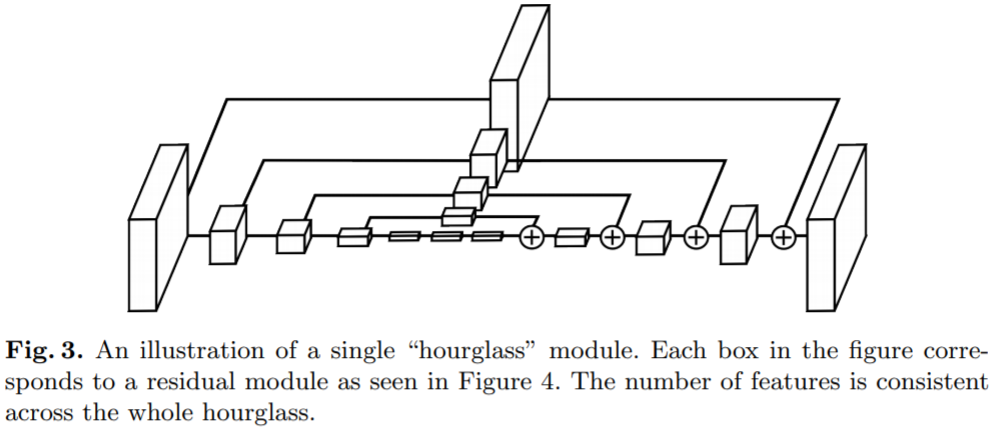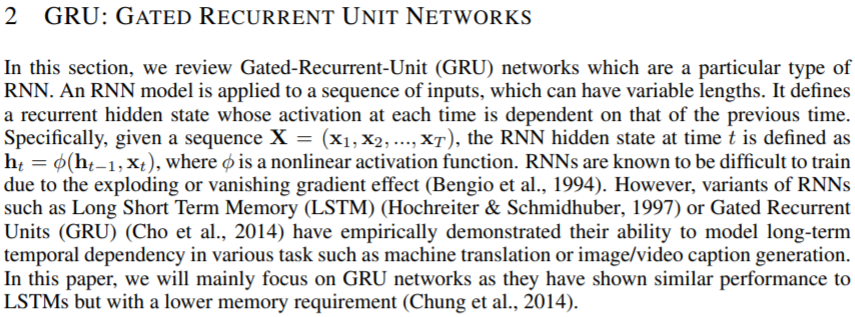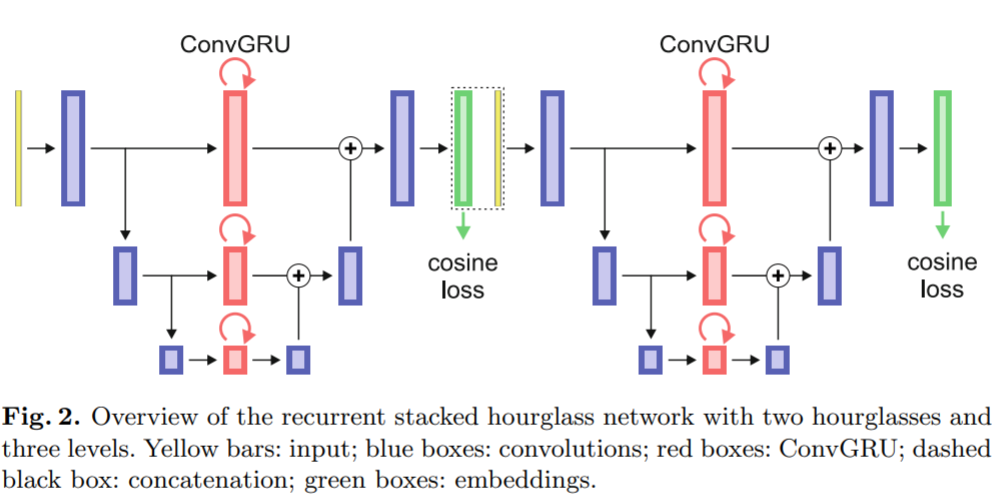# Instance Segmentation with Cosine Embeddings

##### Posted on Apr 25, 2021 (Update: May 24, 2021) 0 Comments

The instance segmentation is more difficult than semantic segmentation, since it does not only assign class labels to pixels, but also distinguishes between instances within each class.

Many methods for instance segmentation have in common that they solely segment one instance at a time. It can be problematic when hundreds of instances are visible in the image.

Recent methods are segmenting each instance simultaneously, by predicting embeddings for all pixels at once. These embeddings have similar values within an instance, but differ among instances.The paper proposes to use recurrent fully convolutional networks for embedding-based instance segementation and tracking, and integrate convolutional gated recurrent units (ConvGRU) into a stacked hourglass network to memorize temporal information.

## Recurrent Stacked Hourglass Network

The paper modifies the stacked hourglass architectureby integrating ConvGRUto propagate temporal information, as shown in the following figure,The hourglass network is similar to the U-Net, i.e., it consists of convolution layers in a contracting and an expanding path for multiple levels, but it additionally introduces convolution layers in the split connections as a parallel path.

The paper exchnage these convolution layers by ConvGRUs, which allow to propagate temporal video information within a fully convolutional network architecture. Each of the ConvGRUs has its own internal state $s_t$ at timestamp $t$, which has a size that is equal to the size of its input.

For $t=0$ the state $s_t$ is initialized with zeros. Based on its current input and current value, the internal state $s_t$ is updated to the new state $s_{t+1}$ after each timestamp. Thus, by consecutively providing the recurrent stacked hourglass network (RSHN) with individual frames, information from previous frames is encoded in the current state and propagated to the next frame.

## Cosine Embedding Loss

Let the network predict a $d$-dimensional embedding vector $e_p\in \IR^d$ for each pixel $p$ of the image. To separate instances $i\in I$,

• firstly, embeddings of pixels $p\in S^{(i)}$ belonging to the same instance $i$ need to be similar,
• secondly, embeddings of $S^{(i)}$ need to be dissimilar to embeddings of pixels $p\in S^{(j)}$ of other instances $j\neq i$.

Treat background as an independent instance. Following from the four color map theorem, only neighboring instances need to have different embeddings. Thus, relax the need of dissimilarity between different instances only to the neighboring ones, i.e., $N^{(i)} = \cup_j S^{(j)}$ for all instances $j\neq i$ within pixel-wise distance $r_N$ to instance $i$. This relaxation simplifies the problem by assigning only a limited number of different embeddings to a possibly large number of different instances.

Define the cosine embedding loss as

$L = \frac{1}{\vert I\vert}\sum_{i\in I}\left(1-\frac{1}{\vert S^{(i)}\vert}\sum_{p\in S^{(i)}}\cos(\bar e^{(i)}, e_p)\right) + \left(\frac{1}{\vert N^{(i)}\vert}\sum_{p\in N^{(i)}}\cos(\bar e^{(i)}, e_p)^2\right)\,.$

By minimizing $L$,

• the first term urges $e_p$ of pixels $p\in S^{(i)}$ to have the same direction as the mean $\bar e^{(i)}$
• the second term pushes $e_p$ of pixels $p\in N^{(i)}$ to be orthogonal to the mean $\bar e^{(i)}$

## Clustering of Embeddings

Since the number of instances is not known, the paper performs grouping with the clustering algorithm HDBSCAN that estimates the number of clusters automatically.

Two parameters for HDBSCAN:

• minimal points $m_{pts}$
• minimal cluster size $m_{clSize}$

To simplify clustering and still be able to detect splitting of instances, cluster only overlapping pairs of consecutive frames at a time. (??)

Note that the embedding loss allows same embeddings for different instances that are far apart, the paper use both image coordinates and value of the embeddings as data points for the clustering algorithm.

Identify same instances by the highest intersection over union (IoU) between each segmented instance in the overlapping frame.

Published in categories Note